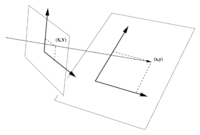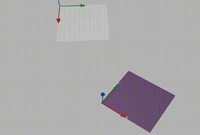# Rotate a plane onto another plane

#### markraz

##### Junior Member
Hi, I have two planes in form of ax+by+cz + d = 0
I need too rotate one plane so it is the same as another plane

what techniques are commonly used to accomplish this?

thanks

#### Subhotosh Khan

##### Super Moderator
Staff member
Hi, I have two planes in form of ax+by+cz + d = 0
I need too rotate one plane so it is the same as another plane

what techniques are commonly used to accomplish this?

thanks
I am not sure about the objective of this question.

Visually, I would calculate the direction cosines of those two planes.

Now I can rotate the "movable" plane about the axes such that the direction cosines of this plane becomes same as the fixed plane.

Now I will have two parallel planes - are those same? May be!!

#### markraz

##### Junior Member
I am not sure about the objective of this question.

Visually, I would calculate the direction cosines of those two planes.

Now I can rotate the "movable" plane about the axes such that the direction cosines of this plane becomes same as the fixed plane.

Now I will have two parallel planes - are those same? May be!!
Thanks for the reply . I this specifically what you mean: https://en.wikipedia.org/wiki/Direction_cosine ??

Which gets me thinking.... Let's say one of my planes was a standard Cartesian coordinate system in the form the following vectors
<0,0,1> <1,0,1> and <0,1,0>. And let's say I have 2 or 3 known vectors that make up the other plane. (the vectors I used to generate the plane equation) Could I just find the angle between these sets of vectors and then rotate the plane based on the differnce in angles?

Ultimatley what I'm trying to do is a take a plane and rotate into the normal xyz plane

#### pka

##### Elite Member
Hi, I have two planes in form of ax+by+cz + d = 0
I need too rotate one plane so it is the same as another plane
what techniques are commonly used to accomplish this?
To markraz, when I first read this post I thought "that's not right". You see, I taught vector space geometry for twenty+ years. In that time I reviewed many textbooks. I never saw any discussion of rotating a plane in a single one of them. Perhaps you can explain what you have in mind here.
Planes are unique sets of points. In the example you gave, $$\displaystyle ax+by+cz+d=0$$ is a unique plane. Its normal vector is $$\displaystyle <a,b,c>$$ which is perpendicular to any line in that plane. If two planes with parallel normals are parallel. If we change the direction of the normal the we change the 'tilt' of the plane. Here is a very readable textbook: A VECTOR SPACE APPROACH TO GEOMETRY by M Hausner.

•markraz

#### markraz

##### Junior Member
To markraz, when I first read this post I thought "that's not right". You see, I taught vector space geometry for twenty+ years. In that time I reviewed many textbooks. I never saw any discussion of rotating a plane in a single one of them. Perhaps you can explain what you have in mind here.
Planes are unique sets of points. In the example you gave, $$\displaystyle ax+by+cz+d=0$$ is a unique plane. Its normal vector is $$\displaystyle <a,b,c>$$ which is perpendicular to any line in that plane. If two planes with parallel normals are parallel. If we change the direction of the normal the we change the 'tilt' of the plane. Here is a very readable textbook: A VECTOR SPACE APPROACH TO GEOMETRY by M Hausner.
Hi Thanks for the reply. Ultimately what I am looking to do is rotate "a set of points" that lie on a plane and rotate these set of points so they lie on another plane. here is a pic#### pka

##### Elite Member
Hi Thanks for the reply. Ultimately what I am looking to do is rotate "a set of points" that lie on a plane and rotate these set of points so they lie on another plane. here is a pic

View attachment 14706
Ah! I now understand. You see, you are using the wrong word. Rotating has nothing to do with the diagram you posted.
But you have not described exactly what the aim is. I frankly am not prepared to give you lessons in these topics.
In addition to the textbook recommended above. Here is another: Modern Geometries by James R. Smart.
You will need to do some real work to understand how to do what is pictured.

#### markraz

##### Junior Member
Ah! I now understand. You see, you are using the wrong word. Rotating has nothing to do with the diagram you posted.
But you have not described exactly what the aim is. I frankly am not prepared to give you lessons in these topics.
In addition to the textbook recommended above. Here is another: Modern Geometries by James R. Smart.
You will need to do some real work to understand how to do what is pictured.
Thanks, I have actually done this several ways using using rodrigues' rotation formula around the normal, or using quaternions around the normal, but that is only useful if I know the "vector equation" of the plane. I was looking for alternative methods using the "standard equation" of the plane without out having any known ortho normnal vectors on the plane. Not sure if this is "projection" since that would not ensure the nominal distance relationships between original points. Thanks I'll check out that book

#### LCKurtz

##### Full Member
I'm still trying to understand what you want to do. Questions I still have are:
1. Do you want every point in the first plane to move to the other plane in an onto 1-1 fashion?
2. Are you wanting to preserve the scale? Your picture looks to be a different scale.
3. If I have a figure on the first plane would the transformation preserve the figure?
4. Is what you want just a linear transformation plus a translation?

#### markraz

##### Junior Member
I'm still trying to understand what you want to do. Questions I still have are:
1. Do you want every point in the first plane to move to the other plane in an onto 1-1 fashion?
2. Are you wanting to preserve the scale? Your picture looks to be a different scale.
3. If I have a figure on the first plane would the transformation preserve the figure?
4. Is what you want just a linear transformation plus a translation?
Hi thanks, correct, that pic is not to scale but I would like to translate/transform the points on one plane to another plane while retaining the positional relationships with respect to the other points. Check out this video

By coincidence the bottom plane happens to be <0,0,1> but I woudl liek to know how to rotate a plane onto any plane thanksLast edited:

#### LCKurtz

##### Full Member
I think this link might be of help to you: